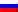# Dijkstra's algorithm

Contenus

## Dijkstra's algorithm

EW Dijkstra (1930-2002) proposed in 1959 an algorithm (called Dijkstra's algorithm) which makes it possible to determine the shortest path between two vertices of a weighted connected graph. Dijkstra's algorithm is based on the following observation: once we determine the shortest path to a vertex v, then the paths that go from v to each of its adjacent vertices might be the shortest path to each of these neighboring peaks. Dijkstra's algorithm is a greedy dynamic programming algorithm, it visits all possible solutions.

Conditions

• No negative length
• Arc or edge
• Finite number of vertices
• A source (and incidentally a target) defined

Dijkstra's algorithm takes as input a directed graph weighted by positive reals and a source vertex. This involves progressively building a subgraph in which the different vertices are classified in increasing order of their minimum distance from the starting vertex. The distance corresponds to the sum of the weights of the borrowed edges.

Summit visited: A summit for which we have determined the shortest route. Once we have defined a vertex as VISIT, this is final, and we will not come back to that vertex.
Peak marked : A summit for which a path has been found. We mark this summit as a CANDIDATE for the shortest path.

Initially, we consider that the distances from each vertex to the starting vertex are infinite except for the starting vertex for which the distance is 0. The starting subgraph is the empty set.

During each iteration, we choose outside the subgraph a vertex of minimum distance and we add it to the subgraph (it becomes a visited vertex). Then, we update the distances of the neighboring vertices of the added one (the vertices are marked). The update takes place as follows: the new distance from the neighboring vertex is the minimum between the existing distance and that obtained by adding the weight of the arc between neighboring vertex and vertex added to the distance from the added vertex.

We continue in this way until the subgraph is completely completed (or until the arrival vertex is selected). Example :

For practical reasons, solving the Dijkstra algorithm only returns a vector containing the visited vertex, the list of predecessors (the vertices already validated) and the values of the shortest paths to all the other vertices. Which corresponds to a current row of the presented table. Thanks to the left column, we can create a tree of the shortest paths from vertex A to all vertices.

Optimality
The starting summit is the shortest way to reach itself. Then, we compute all the size 1 paths starting from this vertex, in order to validate the shortest one. This path is therefore the shortest path from the starting summit because there is no edge of negative weight. By induction, we deduce that the algorithm always validates a shorter path.
```d  = 0, d [i] = infinity for any vertex other than origin
as long as'there is a vertex outside the subgraph P
choose a top To out of P smaller distance d [a]
to put To in P
for each peak b out of P neighbor of To
d [b] = min (d [b], d [a] + weight (a, b))
end
end
return d
```FRFRENRUES